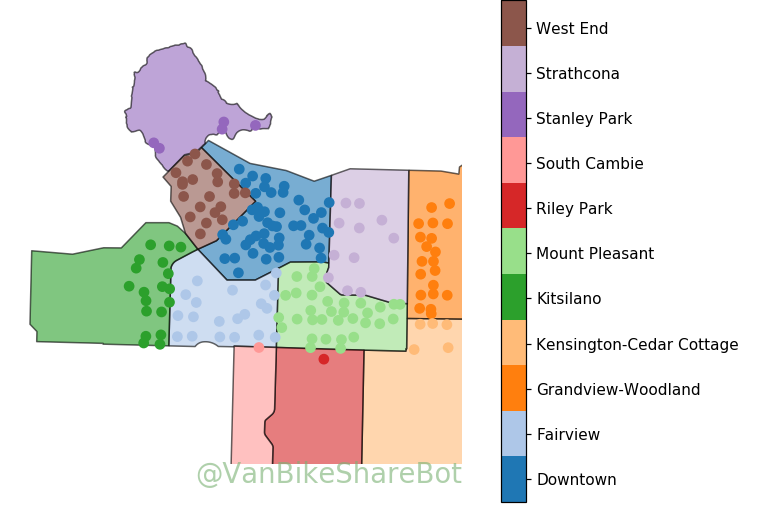# Connecting neighbourhoods

I've been working on how best to look at connections between the various Mobi bikeshare stations throughout Vancouver. One thing the quickly became obvious was that some visualizations are much too noisy with all stations, but work nicely when I group stations into neighbourhoods. Luckily the city geometry files of the various neighourhoods available in their open data collection, so I was able to use those to group the stations according to the city's official definitions. See the distributions of stations below.

In :
```import mobi
import matplotlib as mpl
import matplotlib.pyplot as plt
import numpy as np

import cartopy.crs as ccrs
import shapely
import pyproj

%matplotlib notebook
```
In :
```sdf = mobi.get_stationsdf('/data/mobi/data/')
nhoods = sorted(set(sdf['neighbourhood']))
```
In :
```# Helper function to filter polygons in shapefiles
def shapes_with_atts(shapef,att,vals):
shapes = [s for s,r in sorted(zip(shapes,records),key=lambda x:x.attributes[att]) if r.attributes[att] in vals]
return shapes

# Prep colors. We need sdf.color to be integers (matched to hoods) so that matplotlib can use it with a colormap
color_list = plt.cm.tab20(range(11))
sdf['color'] = sdf.neighbourhood.map({n:c for n,c in zip(nhoods,range(11))})
plot = mobi.GeoPlot()
statlats = sdf.loc[sdf.active,'coordinates'].map(lambda x: x)
statlongs = sdf.loc[sdf.active,'coordinates'].map(lambda x: x)

shapef = '/home/msj/shapes/local_area_boundary.shp'
pshapef = '/home/msj/shapes/park_polygons.shp'
shapes = shapes_with_atts(shapef,'NAME',nhoods)

# Hack to add stanley park in alphabetical order with other neighbourhoods
shapes = shapes[:-2] + shapes_with_atts(pshapef,'PARK_NAME',['Stanley Park']) + shapes[-2:]

s = plot.ax.scatter(statlats,statlongs,transform=ccrs.PlateCarree(),
c=sdf.color,zorder=101,cmap=mpl.colors.ListedColormap(color_list))

plot.f.set_figwidth(7)

cb = plot.f.colorbar(s,fraction=0.35)
cb.set_ticks([(x+0.5)*0.9 for x in range(len(nhoods))])
cb.set_ticklabels(nhoods)
```I've make Stanley Park it's own neighbourhood instead of grouping it in with the West End. There's also only one station each in South Cambie and Riley Park due to a couple of straggler stations south of 16th. The 16th and Heather station is immediately shouth of 16th so could easily be grouped with Fairview but rules are rules.

In :
```import holoviews as hv

from holoviews import opts,dim
from mobi_system_data import *

hv.extension('bokeh',logo=False)
hv.output(size=300)
```